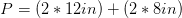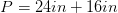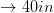# High School Math : Quadrilaterals

## Example Questions

### Example Question #8 : Parallelograms

What is the area of a parallelogram with a base ofand a height of?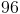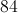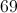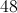Explanation:

To solve this question you must know the formula for the area of a parallelogram.

The formula is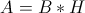So we can plug in the side length for both base and height to yield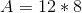Perform the multiplication to arrive at the answer of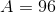.

### Example Question #9 : Parallelograms

Find the area of the following parallelogram: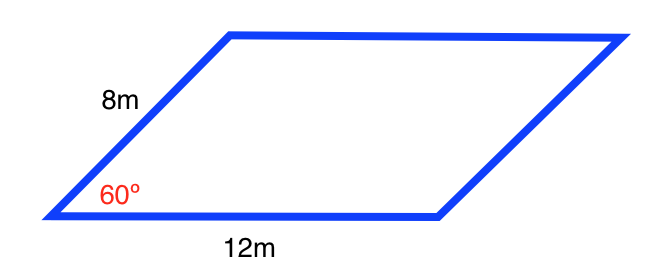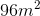Cannot be determined from the given information.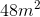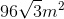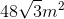Explanation:

The formula for the area of a parallelogram is: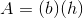,

whereis the length of the base andis the length of the height.

In order to the find the height of the parallelogram, use the formula for a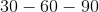triangle: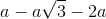, whereis the side opposite the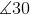.

The left side of the parallelogram forms the followingtriangle: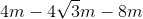, where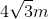is the length of the height.

Plugging in our values, we get: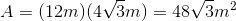### Example Question #10 : Parallelograms

Find the area of the following parallelogram: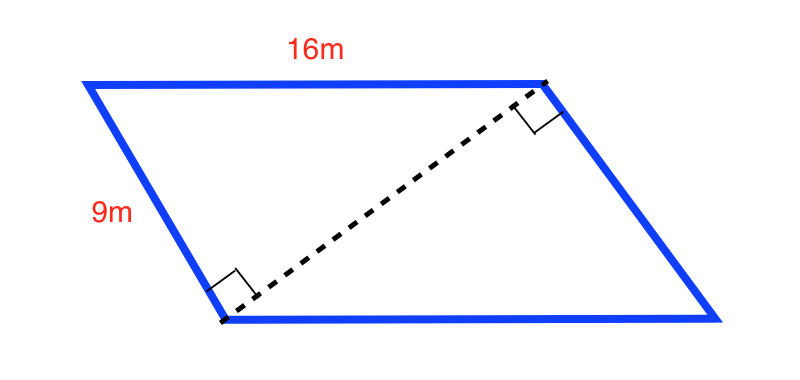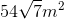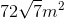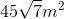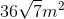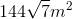Explanation:

Use the Pythagorean Theorem to determine the length of the diagonal: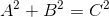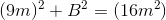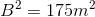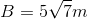The area of the parallelogram is twice the area of the right triangles: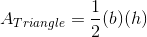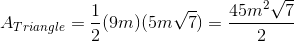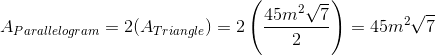### Example Question #31 : Quadrilaterals

Find the area of the following parallelogram: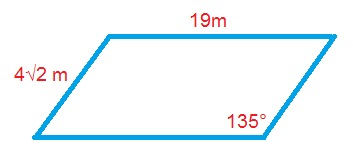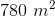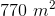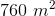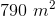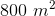Explanation:

The formula for the area of a parallelogram is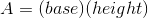.

Use the formula for a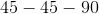triangle to find the length of the height: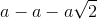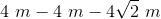Plugging in our values, we get: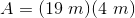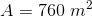### Example Question #1 : How To Find If Rectangles Are Similar

Two rectangles are similar. The perimeter of the first rectangle is 36. The perimeter of the second is 12. If the base length of the second rectangle is 4, what is the height of the first rectangle?

8

4

6

10

2

6

Explanation:

Solve for the height of the second rectangle.

Perimeter = 2B + 2H

12 = 2(4) + 2H

12 = 8 + 2H

4 = 2H

H = 2

If they are similar, then the base and height are proportionally equal.

B1/H1 = B2/H2

4/2 = B2/H2

2 = B2/H2

B2 = 2H2

Use perimeter equation then solve for H:

Perimeter = 2B + 2H

36 = 2 B2 + 2 H2

36 = 2 (2H2) + 2 H2

36 = 4H2 + 2 H2

36 = 6H2

H2 = 6

### Example Question #1 : How To Find The Perimeter Of A Rectangle

A rectangle has a width of 2x. If the length is five more than 150% of the width, what is the perimeter of the rectangle?

6x2 + 5

5x + 5

5x + 10

10(x + 1)

6x2 + 10x

10(x + 1)

Explanation:

Given that w = 2x and l = 1.5w + 5, a substitution will show that l = 1.5(2x) + 5 = 3x + 5.

P = 2w + 2l = 2(2x) + 2(3x + 5) = 4x + 6x + 10 = 10x + 10 = 10(x + 1)

### Example Question #2 : How To Find The Perimeter Of A Rectangle

What is the perimeter of the below rectangle in simplest radical form?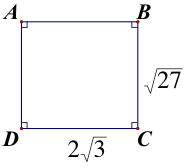4√3 + 2√27

5√3

7√27

10√3

10√3

Explanation:

The perimeter of a figure is the sum of the lengths of all of its sides. The perimeter of this figure is √27 + 2√3 + √27 + 2√3. But, √27 = √9√3 = 3√3 . Now all of the sides have the same number underneath of the radical symbol (i.e. the same radicand) and so the coefficients of each radical can be added together. The result is that the perimeter is equal to 10√3.

### Example Question #7 : How To Find The Perimeter Of A Rectangle

A rectangle has an area of 56 square feet, and a width of 4 feet. What is the perimeter, in feet, of the rectangle?

14
30
36
120
28
Explanation:

Divide the area of the rectangle by the width in order to find the length of 14 feet. The perimeter is the sum of the side lengths, which in this case is 14 feet + 4 feet +14 feet + 4 feet, or 36 feet.

### Example Question #3 : How To Find The Perimeter Of A Rectangle

The length of a rectangle is 3 more inches than its width.  The area of the rectangle is 40 in2.  What is the perimeter of the rectangle?

26 in.

None of the answers are correct

18 in.

34 in.

40 in.

26 in.

Explanation:

Area of rectangle:  A = lw

Perimeter of rectangle:  P = 2l + 2w

w = width and l = w + 3

So A = w(w + 3) = 40 therefore w2 + 3w – 40 = 0

Factor the quadratic equation and set each factor to 0 and solve.

w2 + 3w – 40 = (w – 5)(w + 8) = 0 so w = 5 or w = -8.

The only answer that makes sense is 5.  You cannot have a negative value for a length.

Therefore, w = 5 and l = 8, so P = 2l + 2w = 2(8) + 2(5) = 26 in.

### Example Question #4 : How To Find The Perimeter Of A Rectangle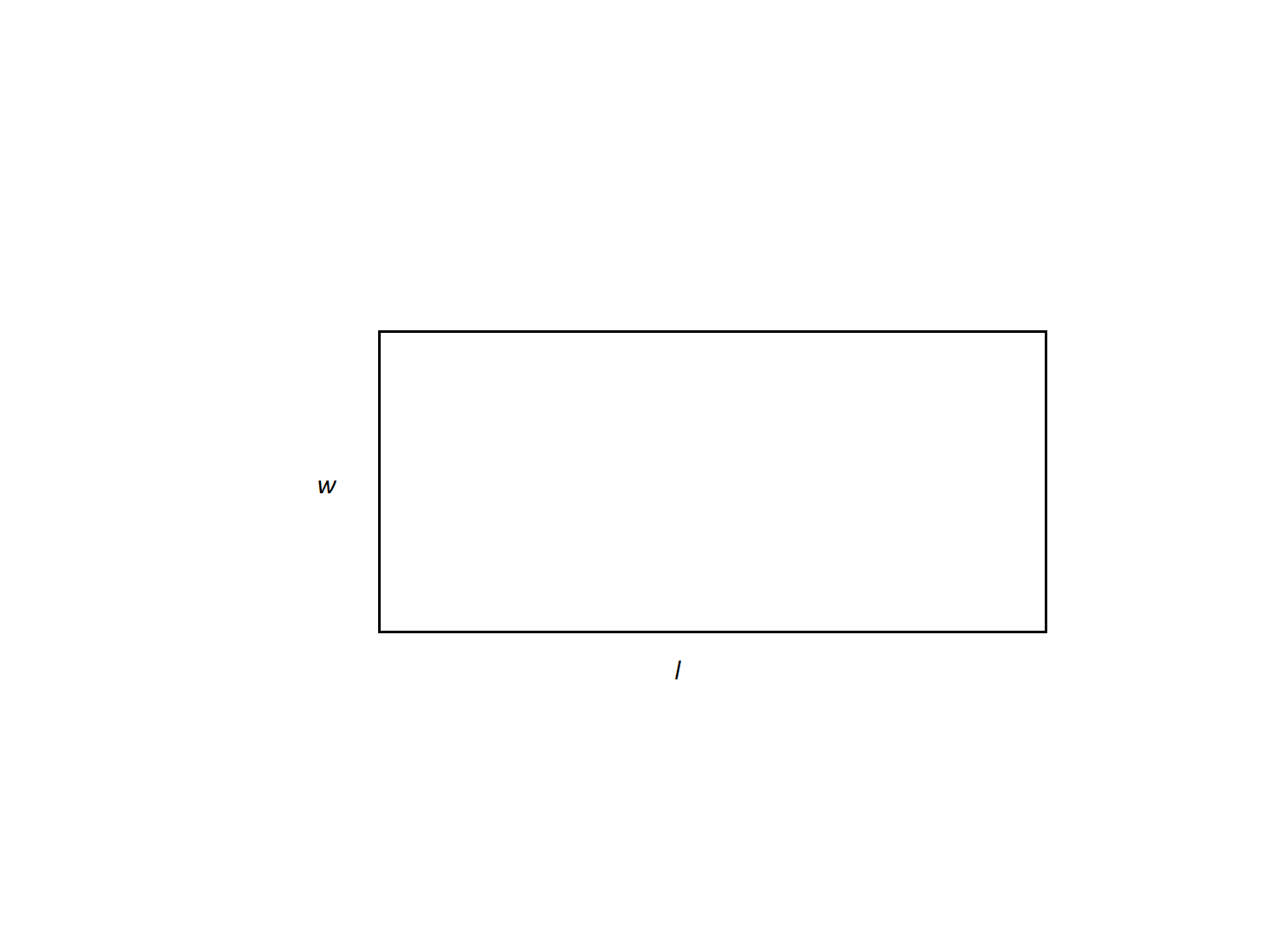The length of a rectangle is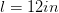and the width is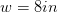. What is its perimeter?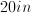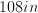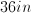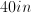Not enough information to solveExplanation:

Perimeter of a quadrilateral is found by adding up the lengths of its sides. The formula for the perimeter of a rectangle is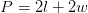.